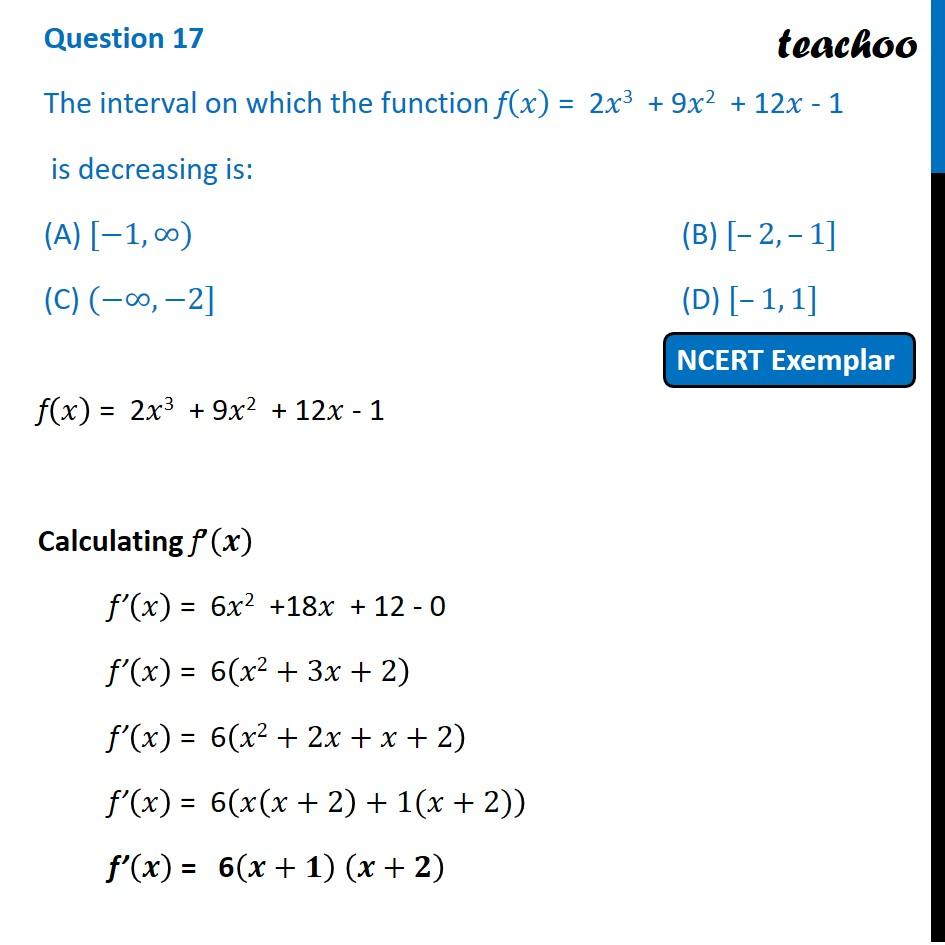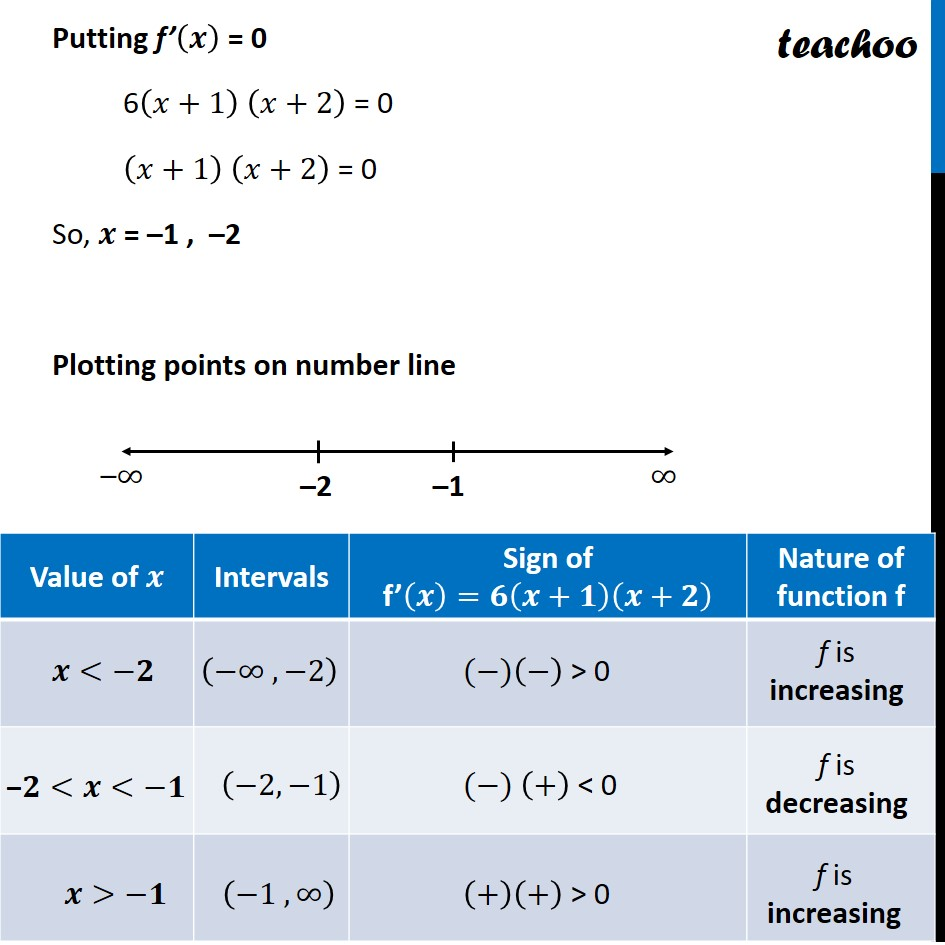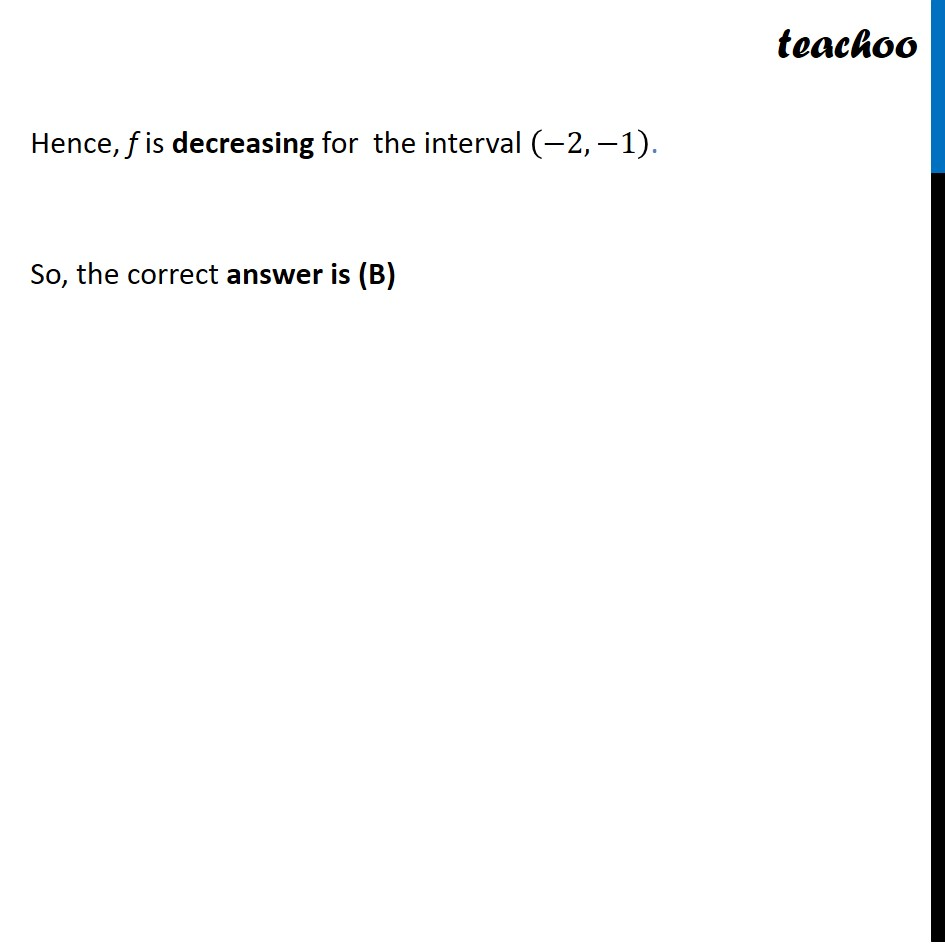NCERT Exemplar - MCQs

Chapter 6 Class 12 Application of Derivatives
Serial order wise

## (D) [–1, 1]

This question is similar to Ex 6.2, 6 - Chapter 6 Class 12 - Application of Derivatives

##googletag.cmd.push(function() { googletag.display('div-gpt-ad-1669298377854-0'); });Get live Maths 1-on-1 Classs - Class 6 to 12

### Transcript

Question 17 The interval on which the function f(𝑥) = 2𝑥3 + 9𝑥2 + 12𝑥 - 1 is decreasing is: (A) [−1,∞) (B) [–2, –1] (C) (−∞,−2] (D) [–1, 1] f(𝑥) = 2𝑥3 + 9𝑥2 + 12𝑥 - 1 Calculating f’(𝒙) f’(𝑥) = 6𝑥2 +18𝑥 + 12 - 0 f’(𝑥) = 6(𝑥2+3𝑥+2) f’(𝑥) = 6(𝑥2+2𝑥+𝑥+2) f’(𝑥) = 6(𝑥(𝑥+2)+1(𝑥+2)) f’(𝒙) = 6(𝒙+𝟏) (𝒙+𝟐) Putting f’(𝒙) = 0 6(𝑥+1) (𝑥+2) = 0 (𝑥+1) (𝑥+2) = 0 So, 𝒙 = –1 , –2 Plotting points on number line Hence, f is decreasing for the interval (−2, −1). So, the correct answer is (B)